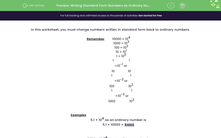# Writing Standard Form Numbers as Ordinary Numbers

In this worksheet, students write standard form numbers as ordinary numbers.Key stage:  KS 3

Curriculum topic:   Number

Curriculum subtopic:   Interpret/Compare Standard Form Numbers

Difficulty level:#### Worksheet Overview

Using standard form is incredibly useful when we either need to use very large or very small numbers.

Other areas we may use standard form includes science. From measuring the distance between stars or the mass of atoms.

Remember

1000 = 103

100 = 102

10 = 101

1= 100

0.1 = 10-1

0.01 = 10-2

0.001 = 10-3

Examples

5.1 × 102 as an ordinary number is

5.1 × 100 = 510

3.214 × 10 -4 as an ordinary number is

3.214 x 0.0001 = 3.214 ÷ 1000 = 0.003214

### What is EdPlace?

We're your National Curriculum aligned online education content provider helping each child succeed in English, maths and science from year 1 to GCSE. With an EdPlace account you’ll be able to track and measure progress, helping each child achieve their best. We build confidence and attainment by personalising each child’s learning at a level that suits them.

Get started